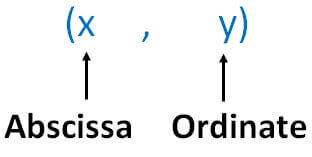Observing points on the graph

Chapter 3 Class 9 Coordinate Geometry
Concept wise

Coordinate of a points isHere, first we write x-coordinate and then y-coordinate

Abscissa is the x-coordinate

Ordinate is the y-coordinate of a point

For example:

Find abscissa and ordinate of (2, 3)

Abscissa = x-coordinate = 2

Ordinate = y-coordinate = 2

Learn in your speed, with individual attention - Teachoo Maths 1-on-1 Class